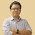Thursday, June 9, 2011

How to Plot Multiple Traces on a StripChart

Written by Erez Wenger - June 2011

In this post we explain, in images and code-lines, how to easily plot multiple traces.

If you are using a stripchart (see our previous stripchart post here) you might want to show two or more traces at once. This can be very useful as you can avoid using many stripcharts, save space, and do it in an elegant way.

To plot multiple traces on a stripchart you need to change the followings:

Changing the number of traces on the UIR
1. Go to the UIR and edit the stripchart control.
2. Click the Traces button.
3. Change the number of traces to the desired amount.
4. Select style and color for each trace.

Changing the number of traces in the code

1. Define an array as large as the amount of traces you want.
2. On each time step calculate the value of each trace.
3. Use the PlotStripChart command to draw the traces.
Example

double y; //Array for 2 traces
switch (event)
{
case EVENT_TIMER_TICK:

y=sin(Pi()*t); //first trace calculation
y=2.0*sin(Pi()*t+0.5); //second trace calculation
PlotStripChart (panelHandle, PANEL_STRIPCHART, y, 2, 0, 0, VAL_DOUBLE); //plotting the traces
t+=0.01;
break;
}

1.Great Job, thank you, Lotfi.

2.Please update pictures in your lessons, thank you very much.

3.Thanks Hung... there is a bug on the website that corrupts images over time. Will be fixed soon.

4.Really helpful, Thanks a lots!

5.super !